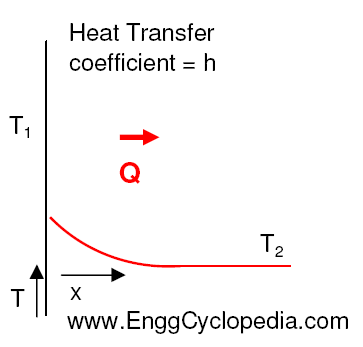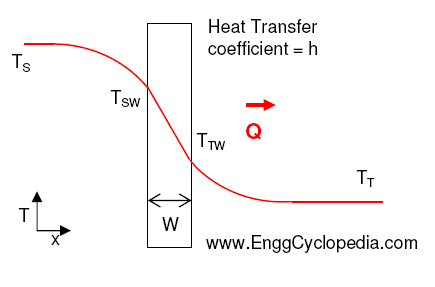# Heat transfer coefficients for heat exchangers

Heat transfer coefficients are a measure of the rate at which heat is transferred between a fluid and a solid surface.  It is the proportionality constant between the heat flux and driving force for the heat transfer which is a temperature difference.

## Heat Transfer Coefficient Units

The units of heat transfer coefficient depend on the type of heat transfer. The most common units are:

• W/m²K (watts per square meter per kelvin) for convection heat transfer coefficient
• W/mK (watts per meter per kelvin) for conduction heat transfer coefficient
• W/m² (watts per square meter) for radiation heat transfer coefficient

## Convective Heat Transfer Coefficient

In a heat exchanger, the convective heat transfer coefficient (h) is a measure of the heat transfer between the fluid and the heat exchanger's surfaces by convection. The value of the convective heat transfer coefficient depends on the properties of the fluid, such as viscosity, density, and thermal conductivity, as well as the flow rate and the surface geometry of the heat exchanger

Steady-state convective heat transfer on either side of a heat exchanger (hot or cold side) can be expressed with the following figure and equation,The steady-state convective heat transfer can be mathematically captured by the following equation,

Q = h×A×(T1 - T2)

where Q: heat transfer rate in Joules/hr
A: Effective heat transfer area of the solid surface in m2
T1 & T2: Steady state temperatures of solid and fluid bodies respectively in 0C
h: Heat transfer coefficient in Joule/(0C×m2)

For heat transfer equipment, separate 'heat transfer coefficients' (h) can be defined for the hot and cold media of the equipment. These heat transfer coefficients can be used to describe the heat transfer on each side of the heat exchanger. In addition, an 'overall heat transfer coefficient' (U) can be defined which describes the overall heat transfer occurring in the exchanger.For example in shell and tube exchangers, heat transfer coefficients on the shell side and tube side describe the heat transfer as,

Q = hS×AS×(TS-TSW) - shell side heat transfer

Q = hT×AT×(TTW-TT) - tube side heat transfer

where Q: heat transfer rate in Joules/hr
AS & AT: Effective heat transfer areas on the shell side and tube side respectively
TS and TSW: Steady state temperatures on shell side fluid and wall surface on shell side in 0C
TT and TTW: Steady state temperatures on tube side fluid and wall surface on tube side in 0C
hS: Shell side heat transfer coefficient in Joule/(0C×m2)
hT: Tube side heat transfer coefficient in Joule/(0C×m2)

In heat exchangers, the convective heat transfer coefficient is usually higher on the fluid side, where the fluid is in direct contact with the heat exchanger's surface, compared to the other side. Correlations can be used to estimate the heat transfer coefficient based on the fluid properties, flow rate, and surface geometry.

## Conductive Heat Transfer Coefficient

In a heat exchanger, the conductive heat transfer coefficient (k) is a measure of the heat transferred through the solid walls of the heat exchanger by conduction.

For steady-state heat transfer in an exchanger, the heat coming to the tube wall through the shell side is equal to the heat transferred through the tube wall by conduction and is equal to the heat going out to the tube side. The conductive heat transfer rate through the tube wall is given by,where N is the tube length and 'r' stands for tube radius. The subscripts 1 and 2 stand for inner and outer tube walls respectively. Tube metal conductivity is expressed as 'k'.

The conductive heat transfer coefficient is a material property and can be determined from the thermal conductivity of the material and the thickness of the heat exchanger walls. Thermal conductivity is a measure of a material's ability to transfer heat and can be found in material properties tables or can be determined experimentally.

## Overall Heat Transfer Coefficient

The overall heat transfer coefficient is defined as the ratio of the heat transfer rate to the log mean temperature difference (LMTD) between the two fluids.

The overall heat transfer in the heat exchanger then may be expressed as,

Q = U×A×(TS-TT) - overall heat transfer rate

U: overall heat transfer coefficient
A: effective overall heat transfer area

Usually, for heat exchanger design, the product UA is expressed as a single entity.

The overall heat transfer coefficient is the reciprocal of the sum of the thermal resistances of the heat exchanger. The thermal resistances are the reciprocal of the convective heat transfer coefficient (h) and the conductive heat transfer coefficient (k) and the resistances due to fouling, radiation, etc. The overall heat transfer coefficient depends on several  factors affecting the individual heat transfer coefficients on both sides (shell side heat transfer coefficient and tube side heat transfer coefficient), as well as conduction in the tube wall.